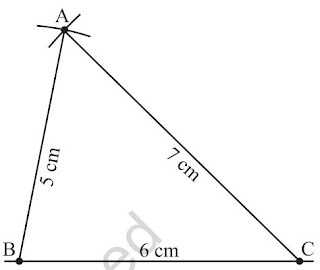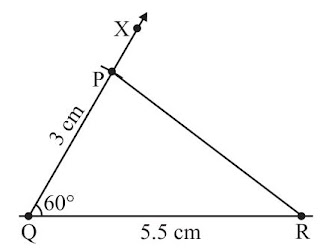>

# Chapter 10 Practical Geometry Class 7 Notes Maths

Chapter 10 Practical Geometry Class 7 Notes Maths will make it easier for the students to comprehend the concepts due to use of easy language. These Revision Notes for Class 7 will be make sure that a student has understood the specifics of every chapter in clear and precise manner. It will ensure that remembering and retaining the syllabus more easy and efficient. A student will enjoy the revising process and make themselves capable of retaining more information so they can excel in the exams. Also, NCERT Solutions for Class 7 Chapter 10 Maths that will be useful in the preparation of exams.• We will learn to draw parallel lines and some types of triangles.

Construction of a line parallel to a given line

• Draw a line segmentland mark a point A not lying on it.
• Take any point B onland join B to A.
• With B as centre and convenient radius, draw an arc cutting at C and AB at D.
• Now with A as centre and the same radius as in above step draw an arc EF cutting AB at G.
• Place the metal point of the compasses at C and adjust the opening so that the pencil point is at D.
• With the same opening as in above step and with Gas centre draw another arc cutting the arc EF at H.
• Now join AH and draw a line m.Construction of Triangles

Important properties concerning triangles

(i) The exterior angle of a triangle is equal in measure to the sum of interior opposite angles.
(ii) The total measure of the three angles of a triangle is 180°.
(iii) Sum of the lengths of any two sides of a triangle is greater than the length of the third side.
(iv) In any right-angled triangle, the square of the length of hypotenuse is equal to the sum of the squares of the lengths of the other two sides.

SSS Criterion

Construct a triangle ABC, given that AB = 5 cm, BC = 6 cm and AC = 7 cm.

• Draw a line segment BC of length 6 cm.
• From B, point A is at a distance of 5 cm. So, with B as centre, draw an arc of radius 5 cm.
• From C, point A is at a distance of 7 cm. So, with C as centre, draw an arc of radius 7 cm.
• A has to be on both the arcs drawn. So, it is the point of intersection of arcs. Mark the point of intersection of arcs as A. Join AB and AC. ∆ABC is now readySAS Criterion

Construct a triangle PQR, given that PQ = 3 cm, QR = 5.5 cm and ∠PQR = 60°.

• Draw a line segment QR of length 5.5 cm.
• At Q, draw QX making 60° with QR. (The point P must be somewhere on this ray of the angle)
• With Q as centre, draw an arc of radius 3 cm. It cuts QX at the point P.
• Join PR. ∆PQR is now obtained.ASA Criterion

Construct ∆XYZ if it is given that XY = 6 cm, m∠ZXY = 30° and m∠XYZ = 100°.

• Draw XY of length 6 cm.
• At X, draw a ray XP making an angle of 30° with XY. By the given condition Z must be somewhere on the XP.
• At Y, draw a ray YQ making an angle of 100° with YX. By the given condition, Z must be on the ray YQ also.
• Z has to lie on both the rays XP and YQ. So, the point of intersection of the two rays is Z. ∆XYZ is now completed.RHS Criterion

Construct ∆LMN, right-angled at M, given that LN = 5 cm and MN = 3 cm.

• Draw MN of length 3 cm.
• At M, draw MX ⊥ MN. (L should be somewhere on this perpendicular)
• With N as centre, draw an arc of radius 5 cm. (L must be on this arc, since it is at a distance of 5 cm from N)
• L has to be on the perpendicular line MX as well as on the arc drawn with centre N. Therefore, L is the meeting point of these two. ∆LMN is now obtained.